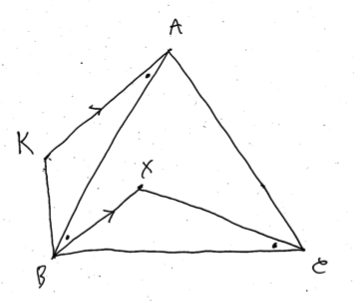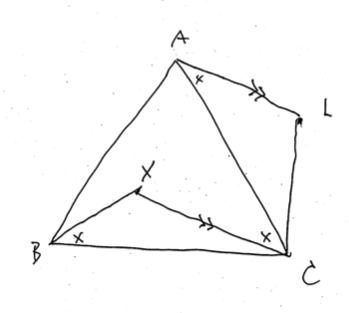# A quiz for a travel in an airplane

Updated:

## Problem

You are given an equilateral triangle $\triangle ABC$ on a plane. Let $X$ be a point inside $\triangle ABC$. Suppose that $\angle BXC = 120^\circ$ and $\bar{BX} : \bar{XC} = 2 : 3$. Calculate the ratio of the aria $\triangle ABX : \triangle ACX$.

## Solution

First, we argue on the side of $B$. Let $K$ be the point that satisfies $\angle XBK = 60^\circ$ and $\bar{BX} = \bar{BK}$. There is two possible points, but see the image below. We see that $\bar{BA} = \bar{BC}$ since $\triangle ABC$ is an equilateral triangle and $\angle KBA = \angle XBC$ since $\angle KBX = \angle ABC = 60^\circ$. Therefore it follows that $\triangle KBA \equiv \triangle XBC$. Thus we have $\angle XCB = \angle KAB$. In addition we have $\angle XBC + \angle XCB = 60^\circ = \angle ABC$. Thus we see that $\angle ABX = \angle KAB$. Hence it follows that $KA // BX$, which implies $\triangle ABX = \triangle KBX$. Note that $\triangle KBX$ is an equilateral triangle.Second, we work the same thing for the side of $C$. Let $L$ be the point that satisfies $\angle XCL = 60^\circ$ and $\bar{CX} = \bar{CL}$. By the same argument, we see that $\triangle ACX = \triangle LCX$ and $\triangle LCX$ is an equilateral triangle.Summing up these result, we conclude that $\triangle ABX : \triangle ACX = \triangle KBX : \triangle LCX = \bar{BX}^2 : \bar{XC}^2 = 4 : 9.$

Categories: